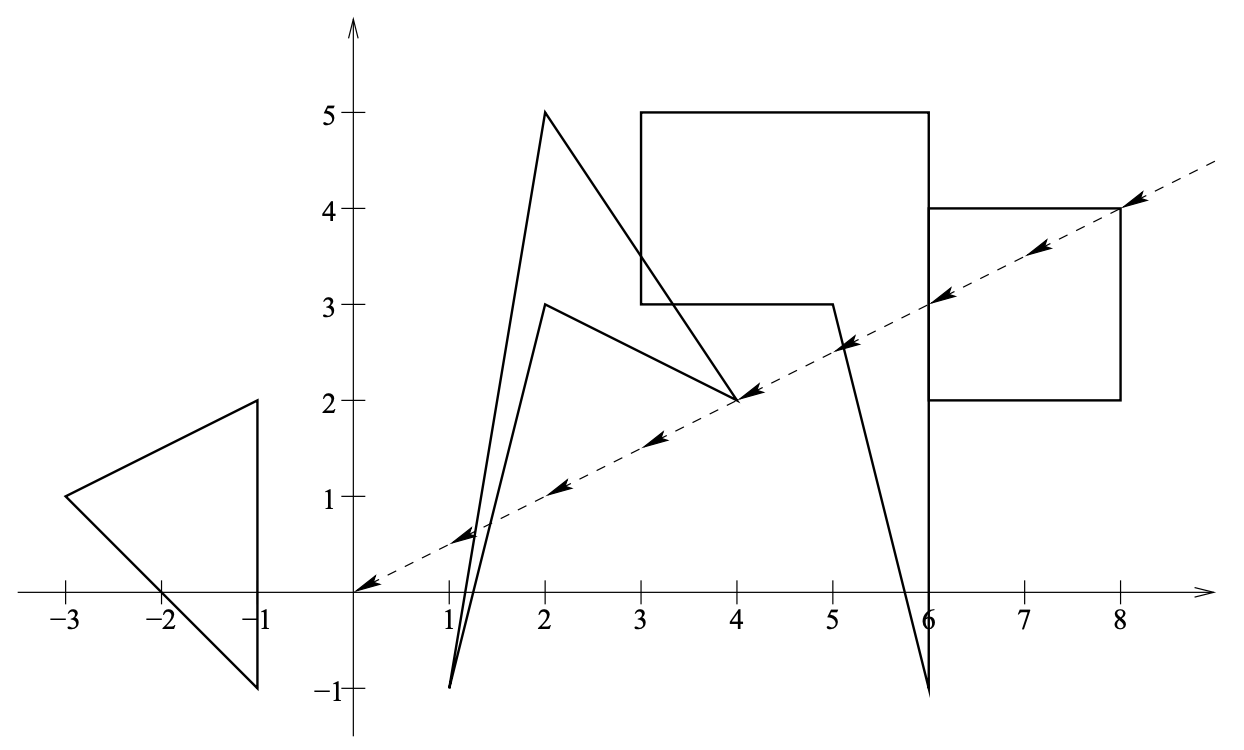시간 제한 메모리 제한 제출 정답 맞은 사람 정답 비율
2 초 128 MB 0 0 0 0.000%

## 문제

There are n clouds in the sky, all moving con with a wind in the same direction and with the same constant velocity v = (vx, vy), i.e. for any real number t ≥ 0 and any point of a cloud with initial coordinates (x, y) the position of this point at time t is (x + t × vx, y + t × vy).

For the sake of simplicity, we assume that every cloud is a polygon (containing its periphery), whose vertices have integer coordinates. This polygon does not have to be convex, but no two of its edges cross each other (with exception of the common endpoints of consecutive edges). The clouds may intersect.

On the ground there is a satellite control center, at coordinates (0, 0), and there is a satellite directly above the control center and above the clouds. Straight upwards from the control center to the satellite goes a laser beam. The laser beam is used to communicate with the satellite. However, when the beam crosses a cloud, the communication is not possible. Initially, the beam does not cross any cloud. During the observation, there may be several moments, when the laser beam crosses (one or more) clouds, interrupting the communication. Even when the laser beam crosses a single vertex of a cloud, the communication is interrupted for an instant. You are to write a program that computes how many times the communication is interrupted, until all the clouds drift away.

Write a program, that:

• reads from the standard input the positions, shapes and velocity of the clouds,
• determines how many times communication is interrupted,
• writes the result to the standard output.

## 입력

The first line of input contains three integers n, v x and vy, separated by single spaces, 1 ≤ n ≤ 1 000, −1 000 000 000 ≤ vx, vy ≤ 1 000 000 000; n is the number of clouds, v = (vx, vy) is the velocity vector of the clouds (v = (0, 0)). The x-coordinates correspond to the West-East direction and the y-coordinates correspond to the North-South direction. The following n lines contain descriptions of the clouds, one cloud per line. Each of these lines consists of a sequence of integers, separated by single spaces.The first integer in a line is the number of cloud’s vertices k, 3 ≤ k ≤ 1 000. It is followed by 2k integers: x1, y1, x2, y2, ..., xk, yk, −1 000 000 000 ≤ xi, yi ≤ 1 000 000 000; (x1, y1), (x2, y2), ..., (xk, yk) are the coordinates of the consecutive vertices in a clockwise order. The laser beam crosses clouds peripheries at most 100 000 times.

## 출력

The first and only line of output should contain exactly one integer: the number of times the communication is interrupted.

## 예제 입력 1

4 -2 -1
4 6 2 6 4 8 4 8 2
4 2 3 1 -1 2 5 4 2
3 -3 1 -1 2 -1 -1
5 5 3 3 3 3 5 6 5 6 -1


## 예제 출력 1

3


## 힌트The figure shows the clouds seen from above. The dashed line marks the points that will cross the laser beam.